19.4 fraction numbers

Fraction numbers describes how many parts of an entire there are. Below the fraction bar is the whole and above the parts of this whole that are actually there. 3/5 means that from the five parts three are actually there.

What is above the bar is called the contatore / numerator and what is under the bar is called the denominatore / denominator. The numerator is no problem, it is just a cardinal number. Except intero and mezzo the denominators doesn't have an own form neither or in other words the ordinal numbers are used.

 summary 1/1 = un intero1/10 = un decimo1/2 = un mezzo 1/12 = un dodicesimo 1/3 = un terzo 1/13 = un tredicesimo 1/4 = un quarto 1/14 = un quattordicesimo 1/5 = un quinto 1/15 = un quindicesimo 1/6 = un sesto 1/16 = un sedicesimo 1/7 = un settimo 1/17 = un diciasettesimo 1/8 = un ottavo 1/18 = un diciottesimo 1/9 = un nono 1/19 = un dicinuovesimo

If the numeratore is bigger than one the denominatore obviously must be in plural.

 5/2 = cinque mezza7/4 = sette quarti37/5 = trentasette quinti214/343 = duecentoquattordici trecentoquarantatresimicontact privacy statement imprint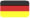GERMAN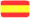SPANISH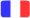FRENCH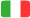ITALIAN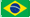PORTUGUESE